## Plus Blog

December 19, 2013

The dramatic curved surfaces of some of the iconic buildings created in the last decade, such as 30 St Mary's Axe (AKA the Gherkin) in London, are only logistically and economically possible thanks to mathematics. Curved panels of glass or other material are expensive to manufacture and to fit. Surprisingly, the curved surface of the Gherkin has been created almost entirely out of flat panels of glass — the only curved piece is the cap on the very top of the building. And simple geometry is all that is required to understand how.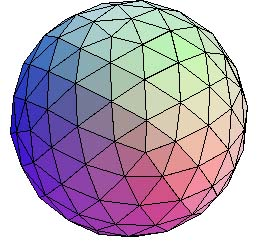A geodesic sphere.

One way of approximating a curved surface using flat panels is using the concept of geodesic domes and surfaces. A geodesic is just a line between two points that follows the shortest possible distance — on the Earth the geodesic lines are great circles, such as the lines of longitude or the routes aircraft use for long distances. A geodesic dome is created from a lattice of geodesics that intersect to cover the curved surface with triangles. The mathematician Buckminster Fuller perfected the mathematical ideas behind geodesic domes and hoped that their properties — greater strength and space for minimum weight — might be the future of housing.

To try to build a sphere out of flat panels, such as a geodesic sphere, you first need to imagine an icosahedron (a polyhedron made up of 20 faces that are equilateral triangles) sitting just inside your sphere, so that the points of the icosahedron just touch the sphere's surface. An icosahedron, with its relatively large flat sides, isn't going to fool anyone into thinking it's curved. You need to use smaller flat panels and a lot more of them.

Divide each edge of the icosahedron in half, and join the points, dividing each of the icosahedron's faces into four smaller triangles. Projecting the vertices of these triangles onto the sphere (pushing them out a little til they two just touch the sphere's surface) now gives you a polyhedron with 80 triangular faces (which are no longer equilateral triangles) that gives a much more convincing approximation of the curved surface of the sphere. You can carry on in this way, dividing the edges in half and creating more triangular faces, until the surface made up of flat triangles is as close to a curved surface as you would like.

Find out more about the Gherkin and other iconic buildings on Plus.

December 18, 2013

How would you go about adding up all the integers from 1 to 100? Tap them into a calculator? Write a little computer code? Or look up the general formula for summing integers?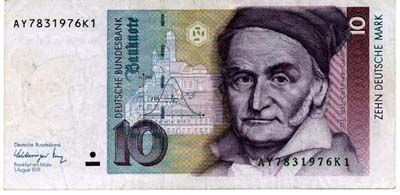Carl Friedrich Gauss as depicted on the (now defunct) German 10 Marks note.

Legend has it that the task of summing those numbers was given to the young Carl Friedrich Gauss by his teacher at primary school, as a punishment for misbehaving. Gauss didn't have a calculator or computer, no one did at that time, but he came up with the correct answer within seconds. Here's how he did it.

Notice that you can sum the numbers in pairs, starting at either end. First you add 1 and 100 to get 101. Next it's 2 and 99, giving 101 again. The same for 3 and 98. Continuing like this, the last pair you get is 50 and 51 and they give 101 again. Altogether there are 50 pairs all adding to 101, so the answer is 50 x 101 = 5050. Easy — if you're Gauss.

December 17, 2013

Sequences of numbers can have limits. For example, the sequence 1, 1/2, 1/3, 1/4, ... has the limit 0 and the sequence 0, 1/2, 2/3, 3/4, 4/5, ... has the limit 1.

But not all number sequences behave so nicely. For example, the sequence 1/2, 1/3, 2/3, 1/4, 3/4, 1/5, 4/5, ... keeps jumping up and down, rather than getting closer and closer to one particular number. We can, however, discern some sort of limiting behaviour as we move along the sequence: the numbers never become larger than 1 or smaller than 0. And what's more, moving far enough along the sequence, you can find numbers that get as close as you like to both 1 and 0. So both 0 and 1 have some right to be considered limits of the sequence — and indeed they are: 1 is the limit superior and 0 is the limit inferior, so-called for obvious reasons.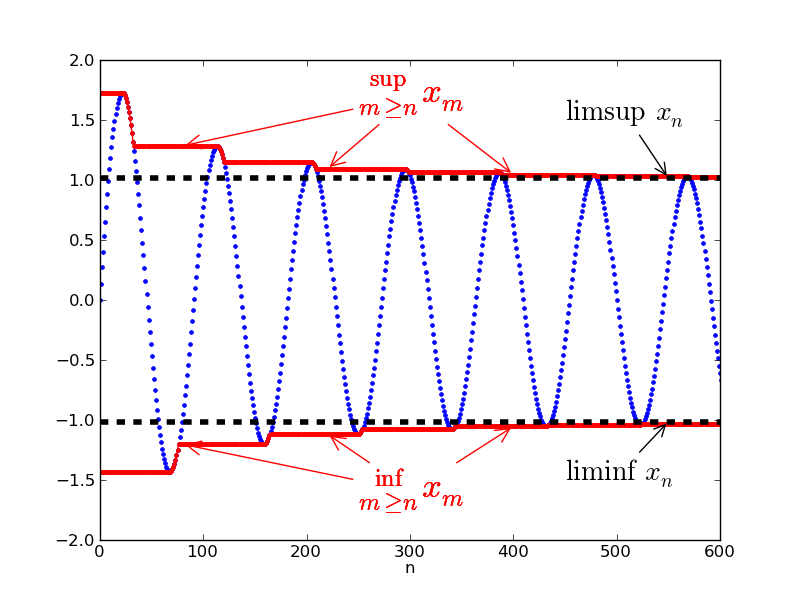But can you define these limits superior and inferior for a general sequence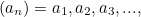for example the one shown in the picture? Here’s how to do it for the limit superior. First look at the whole sequence and find its least upper bound: that’s the smallest number that’s bigger than all the numbers in the sequence. Then chop off the first number in the sequence,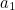, and again find the least upper bound for the new sequence. This might be smaller than the previous least upper bound (if that was equal to), but not bigger. Then chop off the first two numbers and again find the least upper bound.

Keep going, chopping off the first three, four, five, etc numbers, to get a sequence of least upper bounds (indicated by the red curve in the picture). In this sequence every number is either equal to or smaller than the number before. The limit superior is defined to be the limit of these least upper bounds. It always exists: since the sequence of least upper bounds is either constant or decreasing, it will either approach minus infinity or some other finite limit. The limit superior could also be equal to plus infinity, if there are numbers in the sequence that get arbitrarily large.

The limit inferior is defined in a similar way, only that you look at the sequence of greatest lower bounds and then take the limit of that.

You can read more about the limits inferior and superior in the Plus article The Abel Prize 2012.

December 16, 2013

Here's a dilemma. Suppose you and a friend have been arrested for a crime and you're being interviewed separately. The police offer each of you the same deal. You can either confess, incriminating your partner, or remain silent. If you confess and your partner doesn't, then you get 2 years in jail (as a reward for talking), while your partner gets 10 years. If you both confess, then you both get 8 years (reduced from 10 years because at least you talked). If you both remain silent, you both get 5 years, as the evidence is only sufficient to convict you of a lesser crime.Solitary confinement

What should your strategy be? As a selfish and rational individual, you should talk. If your partner also talks, then your confession gets you 8 years instead of 10. If your partner doesn't talk, then it gets you 2 years instead of 5. Talking is your dominant strategy, it leaves you better off than silence, no matter what your partner does.

The trouble is that your partner, just as selfish and rational as you, will come to the same conclusion. You'll both decide to talk and get 8 years each. Paradoxically, your dominant strategy will leave both of you worse off than silence would have done.

The prisoner's dilemma is one of game theory's most famous games because it illustrates why people might refuse to cooperate when they would be better off doing so. One real-life situation that is similar to the dilemma is an arms race between two countries, in which both countries increase their military might when it would be better for both to disarm.

The dilemma has been used extensively in mathematical research into altruism. Mathematical research into altruism? Yes, that's right! Using the dilemma as the basis for computer simulations in which simulated individuals can either cooperate or defect has shown how altruism can evolve as a survival strategy, even in large societies.

To find out more, read Does it pay to be nice? And there's more about the prisoner's dilemma and economics in Adam Smith and the invisible hand.

December 15, 2013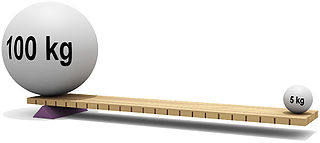Image © 2004 César Rincón.

Kneeling in the mud by a country road on a cold drizzly day recently, we finally appreciated the wonder that is a lever. We were trying to change a flat tyre and even jumping on the end of the wheel wrench wouldn't budge the wheel nuts. But when the AA arrived they undid them with ease, thanks to a wheel wrench that was three times the size of ours. There you have it ... size really does matter!

A lever is a truly remarkable device that can literally give any of us the strength of ten men. You can counteract 10 men pushing down on one side of a see-saw by applying just 1/10th of their force, as long as you are 10 times further from the see-saw's centre as they are. This is because the forces acting on a lever are proportional to the distances they are from the fulcrum. In this way a small amount of force moving a longer distance can move a large load over a smaller distance.

Levers are working hard all around us: in see-saws (where the fulcrum is between the loads), in wheel barrows (where the load is between the fulcrum and the force) and even in our very jaws (where the force is applied between the fulcrum and the load).

Archimedes was the first to mathematically describe how levers work and famously said: "Give me fulcrum and I shall move the Earth with a lever." And give us a long enough wheel wrench and we might just be able to change my next flat tyre for ourselves!

You can read more about mechanics on Plus.

December 14, 2013

A polyhedron is the 3D version of a polygon. It's a solid object whose surface is made up of a number of polygonal faces. Two faces meet in an edge and the corners of a polyhedron are called vertices.

Euler's polyhedron formula, named after Leonhard Euler, is a pretty amazing equation relating the number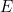of edges,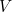of vertices and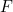of faces of a polyhedron: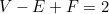As an example, think of a cube. It's got 6 faces, 8 vertices and 12 edges: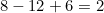as required.

The amazing thing is that this formula holds for all polyhedra, except for those that have holes running through them.

Using this formula, you can figure out quickly that there is no simple polyhedron (that is one without holes) with exactly seven edges. Similarly, there is no simple polyhedron with ten faces and seventeen vertices.

The formula also makes it possible to prove one of the most beautiful results in geometry, that there are only five Platonic solids.Figure 7: The Platonic solids. From left to right we have the tetrahedon with four faces, the cube with six faces, the octahedron with eight faces, the dodecahedron with twelve faces, and the icosahedron with twenty faces.

Euler's polyhedron formula applies to solids that, in a topological sense, are equivalent to the sphere: you can turn each simple polyhedron into a sphere by smoothing out the edges and corners and making it round. But you can also look at the number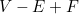for polyhedra that are topologically the same as a doughnut, or a doughnut with two, three, four or any number of holes. This number is called the Euler characteristic and it turns out that it is always equal to 2 minus 2 times the number of holes. So the Euler characteristic doesn't only tell us something about polyhedra, but also something very deep about the nature of shape and space in general.

You can find out more about Euler's polyhedron formula, including a proof, in this Plus article. And there's an interesting application to designing footballs in A fly walks around a football.